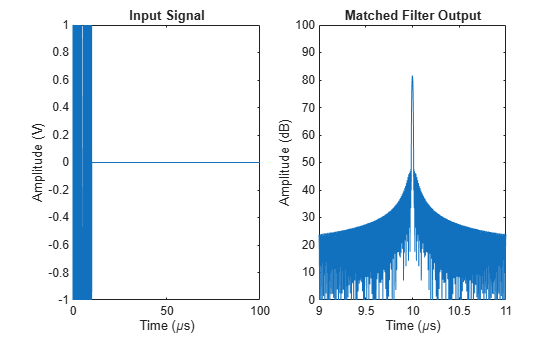# nlfmspec2freq

Instantaneous frequency of nonlinear frequency-modulated waveform

Since R2023a

## Syntax

``freq = nlfmspec2freq(BW,S)``

## Description

example

````freq = nlfmspec2freq(BW,S)` returns samples of the instantaneous frequency `freq` for a nonlinear frequency modulated (NLFM) pulse waveform. The waveform sweeps the bandwidth `BW` and has a power spectrum shape `S`. The frequency values in `freq` are found by applying the principle of stationary phase to the power spectrum shape `S`.```

## Examples

collapse all

Create a nonlinear FM waveform derived from a power spectral density function shaped as a Taylor window with -35 dB sidelobes. The pulse bandwidth is 120 MHz and the pulse duration is$10\mu$sec. Generate matched filter coefficients and then apply a matched filter. Plot the resulting matched filter output to display the range sidelobe levels.

```BW = 120e6; T = 10e-6; fs = 10*BW;```

Generate 200 points of a waveform with instantaneous frequency values defined by a Taylor window. The window has -35 dB sidelobe levels.

```w = taylorwin(200,4,-35); freq = nlfmspec2freq(BW,w); waveform = phased.CustomFMWaveform('SampleRate',fs, ... 'PulseWidth',T,'FrequencyModulation',freq, ... 'OutputFormat','Pulses','CoefficientsOutputPort',true); disp(['Bandwidth = ',num2str(bandwidth(waveform)/1e6),' MHz'])```
```Bandwidth = 119.9644 MHz ```

Obtain the matched filter coefficients from the waveform.

```[wav,coeff] = waveform(); filter = phased.MatchedFilter('CoefficientsSource','Input port'); mfout = filter(wav,coeff);```

Plot input signal and matched filter output.

```t = (0:numel(wav)-1)/fs; figure subplot(121) plot(t*1e6,real(wav)) xlabel('Time (\mus)') ylabel('Amplitude (V)') title('Input Signal') subplot(122) plot(t*1e6,mag2db(abs(mfout))) xlabel('Time (\mus)') ylabel('Amplitude (dB)') title('Matched Filter Output') xlim([9 11]) ylim([0 100])```## Input Arguments

collapse all

Pulse waveform bandwidth, specified as a positive scalar. Units are in Hz.

Example: `10e3`

Data Types: `double`

Power spectrum shape, specified as a real-valued vector. Units are dimensionless.

Data Types: `double`

## Output Arguments

collapse all

Instantaneous waveform frequencies, returned as a real-valued row vector. The instantaneous frequencies form a single up-sweep. `freq` has the same number of elements as `S`. Units are Hz.

Data Types: `double`

 Collins, T., and P. Atkins. "Nonlinear frequency modulation chirps for active sonar" IEE Proceedings-Radar, Sonar and Navigation 146.6 (1999): 312-316.

 Levanon, Nadav, and Eli Mozeson. Radar signals. John Wiley & Sons, 2004, pp. 92-93.

 Doerry, Armin Walter. "Generating nonlinear FM chirp waveforms for radar". No. SAND2006-5856. Sandia National Laboratories (SNL), Albuquerque, NM, and Livermore, CA (United States), 2006.

 Cook, C. E. "A class of nonlinear FM pulse compression signals." Proceedings of the IEEE 52.11 (1964): 1369-1371.